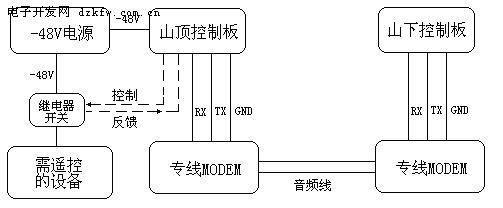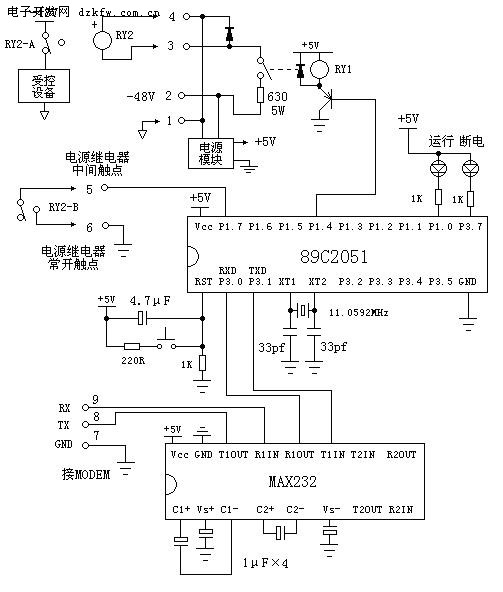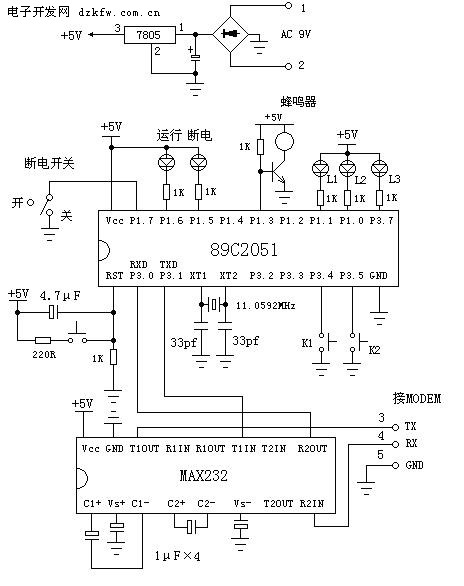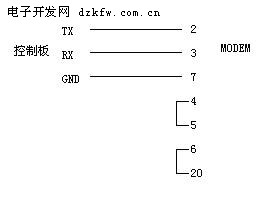# 采用单片机实现远程电源控制

我单位有一无人值守的机房位于一高山山顶上，上山的路是600多级的台阶。因通信需要，有时要开关某些机器设备，操作上虽然简单，但要工作人员花10多分钟爬一趟山，不仅辛苦，而且拖延了时间。为解决此问题，本人利用89C2051单片机，设计了一遥控开关，在山下机房便可对山顶上的设备进行开关机。

该方案的框图如下：山顶机房及山下机房各安装一块控制板，两者之间通过专线MODEM相连。山下控制板主要功能是：将操作人员的开关信息转换成指令，发送给山顶控制板，并根据山顶控制板发来的电源通断状态报告指令，以指示灯的形式显示给操作人员。山顶控制板主要功能是：通过控制继电器的吸放来控制设备的电源，该板在接收到山下控制板发来的开关电指令后，驱动继电器的吸放，并将继电器的反馈状态转换成指令，报告给山下控制板。两处的专线MODEM由本单位内部的光纤通信设备提供的音频线路连接。

山顶控制板所包括的主要元件有单片机芯片89C2051，电平转换芯片MAX232，电源模块。由于需遥控的通信设备使用-48V电源，山顶控制板也采用-48V。该板采用了一个成品开关电源模块，将-48V转换成+5V。芯片MAX232的功能是把单片机串口的TTL电平转换成MODEM的RS-232电平，使单片机能通过MODEM收发数据。2051单片机根据山下控制板发来的指令，通过P1_4脚控制线圈电压为5V的小继电器RY1的吸放，进而控制电源继电器RY2的吸放。电源继电器RY2为两组触点、24V线圈电压的大继电器，触点可承受较大电流。其中的一组触点用于控制设备电源的通断，接中间触点及常闭触点。继电器释放时，设备加电，继电器吸合时，设备关电。另外一组触点作为继电器动作后的反馈，接中间触点及常开触点，分别接地及2051的P1_7脚。当继电器RY2吸合时P1_7经继电器接地，为低电平，继电器释放时P1_7脚为高平（2051内部有上拉电阻）。单片机2051每秒钟检测一次P1_7脚是否接地，以此判定继电器是否吸合（即是否断开了设备的电源），随后将检测的结果转换成指令，通过MODEM向山下的控制板汇报，同时本身的断电指示灯也显示出设备的加断电状态。
山顶控制板的电路图如下：山顶控制板的程序如下：
#include "atmelAT89X51.H"
#define  SYN  'Z'    //来自山下控制板数据帧的同步字符

char countdown;      //时间计数

char TX_buf;      //发送缓冲区
char TX_len;         //发送字符串长度
char TX_num;

char RX_buf;      //接收缓冲区
char RX_len;         //接收字符串长度
char RX_num;
char CRC(char *buf,char len)        //校验码生成函数
{char i,temp;
temp=0;
for(i=0;i<len;i++)   temp=temp^buf[i];
return(temp);
}

void timer0_int() interrupt 1         //定时器0的中断服务程序
{ TL0=0x00;   TH0=0x0A6;
countdown--;
if(countdown==0 || countdown==20)
P1_0=!P1_0;              //控制CPU运行指示灯的秒闪

if(countdown!=0) return;
countdown=40;   //过了一秒钟
P3_7=P1_7;      //检测电源继电器的吸放状态，并驱动P3_7的指示灯
if(P1_7)  TX_buf=0x13;     //高电平，电源继电器已释放，设备电源接通
else      TX_buf=0x31;    //接地，电源继电器已吸合，设备电源中断
TX_buf=CRC(TX_buf,2);    //生成校验码
TX_num=0;
SBUF=TX_buf;            //向山下控制板报告继电器的吸放状态
}
void serial_int() interrupt 4        //串口中断服务程序
{ if(TI)                        //发送触发了中断
{ TI=0;    TX_num++;
if(TX_num<TX_len)   SBUF=TX_buf[TX_num];
}
else                         //接收触发了中断
{ RI=0;    RX_buf[RX_num]=SBUF;
if(RX_num==0 && RX_buf[RX_num]!=SYN)
return;                  //在接收的数据中搜索同步字符
RX_num++;
if(RX_num==RX_len)       //收完一条指令
{ RX_num=0;
if(RX_buf[RX_len-1]==CRC(RX_buf,RX_len-1)) //检查校验码是否正确
{ if(RX_buf==0x13)   P1_4=1;   //释放小继电器RY1及电源继电器RY2
if(RX_buf==0x31)   P1_4=0;  //吸合小继电器RY1及电源继电器RY2
}
}
}
}

void main()
{  IE=0x92;
TMOD=0x21;               //定时器1：模式2，定时器2：模式1
TL1=253;     TH1=253;     // 9600波特率
TR1=1;                    //启动定时器1
SCON=0x50;                //串口：模式1

TL0=0x00;    TH0=0x0A6;   //定时器0定时0.025秒
TR0=1;                    //启动定时器0
countdown=40;              //1秒=0.025*40
P1_4=1;                    //释放继电器RY1、RY2

TX_buf=0x7E;   TX_len=3;
RX_num=0;        RX_len=3;

while(1) ;
}

山下控制板的电路图如下所示，所包括的主要元件有单片机芯片89C2051，电平转换芯片MAX232，7805稳压芯片。芯片MAX232的功能是把单片机串口的TTL电平转换成MODEM的RS-232电平，使单片机能通过MODEM收发数据。需要对山上设备进行开关电操作时，先把连接在2051单片机P1_7脚的断电开关拨到“开”或“关”的位置，然后连续按下K1按键，直到L1、L2、L3三个操作指示灯全亮，接着按一下K2按键，L1、L2、L3指示灯全灭，此时2051单片机检查P1_7脚的电平，如果是低电平，则向山顶控制板发断电指令，如果是高电平，则向山顶控制板发加电指令。K1、K2的其它按键组合均不使单片机发送加断电指令。这里采取断电开关与按键相结合的控制方式，目的是为了防止意外的开关操作，提高安全性。MODEM通信正常的情况下，山下控制板每秒钟收到一次山顶控制板发来的加断电状态报告。当接收到状态报告后，经单片机分析，如果是断电状态，则P1_5脚输出低电平，点亮断电指示灯，P1_3脚输出高低脉冲，驱动蜂鸣器告警提示；如果是加电状态，断电指示灯灭，蜂鸣器静音。如果连续3秒钟收不到山顶控制板的状态报告，断电指示灯将作秒闪、蜂鸣器告警，提示操作人员检查MODEM线路是否正常。#include "atmelAT89X51.H"
#define  SYN   0x7E   //山顶控制板发来数据帧的同步字符

char countdown;      //时钟计数
char TTL;           //通信中断的时间门坎值，设置为3秒
bit  link_error;      //通信中断标志
bit  power_on;      //山上设备是否加电的标志
bit  km;            //按键消抖动标志
bit  kp;            //按键操作已处理标志
char TTW;          //发送指令前的时间计数

char TX_buf;      //发送缓冲区
char TX_len;         //发送指令长度
char TX_num;         //当前发送的字符序号

char RX_buf;      //接收缓冲区
char RX_len;         //接收指令长度
char RX_num;        //当前接收的字符序号

char CRC(char *buf,char len)    //校验码生成函数
{char i,temp;
temp=0;
for(i=0;i<len;i++)   temp=temp^buf[i];
return(temp);
}

void timer0_int() interrupt 1    //定时器0的中断服务函数
{ bit key1,key2;

TL0=0x00;   TH0=0x0A6;
countdown--;
if(countdown==0 || countdown==20)
{ P1_6=!P1_6;            //CPU运行指示灯秒闪
if(link_error)
P1_5=!P1_5;        //通信中断，断电指示灯秒闪
else
{ if(power_on)   P1_5=1;    //设备加电，断电指示灯灭
else          P1_5=0;    //设备关电，断电指示灯亮
}
}

if(power_on && !link_error)        //当设备加电且通信正常
P1_3=0;                     //关闭蜂鸣器
else                           //当设备断电或通信中断
{ if(countdown==0)   P1_3=0;   //蜂鸣器告警
if(countdown==5)   P1_3=1;
if(countdown==10)  P1_3=0;
if(countdown==15)  P1_3=1;
}

key1=P3_4;  key2=P3_5;
if(key1==1 && key2==1)  {  km=0;kp=0;  }   //两个按键均没有按下
else
{ if(km==0)   km=1;             //设消抖动标志
else
{ if(kp==0)
{ kp=1;
if(key1==0)              //按键K1被按下
TTW=(TTW+1)%4;      //计算K1连续按下的次数
if(key2==0)              //按键K2被按下
{ if(TTW==3)           //如果K1已被连续按了三次
{ if(P1_7)    TX_buf=0x13;    //发加电指令
else        TX_buf=0x31;    //发关电指令
TX_buf=CRC(TX_buf,2);
TX_num=0;   SBUF=TX_buf;
}
TTW=0;    //不管K1已按下几次，K2按下后复位TTW计数器
}
}
}
}

if(countdown!=0)   return;
countdown=40;

if(TTL==0)  link_error=1;    //TTL减到0，表示通信中断
else        TTL--;         //每隔1秒对TTL作减1操作
}

void serial_int() interrupt 4       //串口中断服务程序
{ if(TI)
{ TI=0;    TX_num++;
if(TX_num<TX_len)   SBUF=TX_buf[TX_num];
}
else
{ RI=0;    RX_buf[RX_num]=SBUF;
if(RX_num==0 && RX_buf[RX_num]!=SYN)
return;                   //在接收到的数据中搜索同步字符

RX_num++;
if(RX_num==RX_len)       //接收到一完成指令
{ RX_num=0;
if(RX_buf[RX_len-1]==CRC(RX_buf,RX_len-1))   //检查校验
{ if(RX_buf==0x13)  power_on=1;       //加电状态
if(RX_buf==0x31)  power_on=0;      //断电状态
TTL=3; link_error=0;        //通信正常，重置TTL值
}
}
}
}

void main()
{  IE=0x92;
TMOD=0x21;                  //定时器1：模式2，定时器0：模式1
TL1=253;        TH1=253;     //9600波特率
TR1=1;                       //启动定时器1
SCON=0x50;                   //串口：模式1

TL0=0x00;      TH0=0x0A6;    //定时器0定时0.025秒
TR0=1;                        //启动定时器0
countdown=40;                  //1秒=0.025秒*40

TTL=3;                       //连续3秒收不到报告，表示通信中断
TTW=0;
km=0;         kp=0;
link_error=1;    power_on=1;
TX_buf='Z';  TX_len=3;
RX_num=0;     RX_len=3;

while(1)
{ if(TTW==0)   { P3_7=1; P1_0=1; P1_1=1; }
if(TTW==1)   P3_7=0;
if(TTW==2)   P1_0=0;
if(TTW==3)   P1_1=0;
}
}

单片机2051与MODEM之间的串口通信电缆只用RX、TX、GND三根线，其他的握手信号均没有使用，但在制作MODEM一端的接头时应要按下图制作：##### Tags：单片机,远程,电源,控制

• 上一篇文章：
• 下一篇文章：
• 请文明参与讨论，禁止漫骂攻击，不要恶意评论、违禁词语。 昵称：
 1分 2分 3分 4分 5分 还可以输入 200 个字
网友评论
推荐文章
最新推荐
热门文章